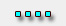﻿ shielding,shielded,welded shielding room,shielding cabinet,shielding table,emc,mri,rf,cnemc当前位置：拓达 >> en >> essays >> 浏览文章Test EMC using novel time-domain methods(1)

By Florian Krug Tobias Hermann Peter Russer

Institute for High-Frequency Engineering Technische Universitaet Muenchen

Due to the rapid advancements in technologies, the ability to achieve EMC has become a major  challenge in developing electronic products. To reduce the cost and improve the quality of circuit   and system development, measurement equipment for EMC and EMI are used to extract extensive and accurate information within short measurement times.

In the past, radio noise and EMI were measured and characterized by superheterodyne radio receivers. The disadvantage of this method is long measurement time—typically 0mins for frequency bands of 30MHz to 1GHz. Since such a long measurement time results in high test costs, it is important to look for other options to reduce measurement time without losing quality. Since conventional measurement systems do not evaluate the phase information of the measured EMI signal, important information is lost. Digital processing of EMI measurements using Fourier transform allows the decomposition of a signal measured in time-domain into its spectral components. The use of Fourier transform techniques has grown rapidly in recent years because of the economy of programs using the FFT.
This article covers new ways of signal processing to use timedomain measurement techniques to perform accurate and time-efficient EMI measurements. Also, signal processing strategies for an accurate measurement of various types of EMI signal are shown.

Time-domain EMI system

The block diagram of the timedomain measurement setup consisting of the time-domain electromagnetic interference measurement (TDEMI) and a conventional EMI receiver is depicted in Figure 1. The EMI receiver is used for comparison. The TDEMI consists of a broadband antenna (HL562, Rohde- &Schwarz), line impedance stabilization network (ESH 2-Z5, Rohde&Schwarz), switching unit (RSU, Rohde-&Schwarz), amplifier (ZFL-1000LN, mini-circuits), lowpass filter (SLP-1000, mini-circuits), ADC (TDS7154, Tektronix and oscilloscope) and a PC (IBM compatible). This system is based on digital processing of sampled EMI signals. One of its advantages is that the performance of the system may be improved via software.

The ADC samples and quantizes the continuous input signal x(t) with a sampling frequency fTest EMC using novel time-domain methods corresponding to a sampling period of 1=fs = Δt. Shannon’s Theorem requires fs to be at least twice as high as the highest signal frequency. The upper limit imposed on the signal frequency by the sampling process is also called Nyquist frequency. After digitization, the data is being delivered to the evaluation routine in blocks of N samples, where it is used as input to the spectral estimator. The mathematical basis for the spectral estimation methods used in the context of the TDEMI system is the discrete Fourier transform (DFT). The DFT being applied to each of the data blocks is defined by Equation 1

The DFT transforms the discrete-time signal sequence x[n] into the discrete-frequency spectral sequence X[r], with n and r denoting the discrete-time response frequency variable:
x[n] = x(nΔt); X[r] = X(rΔf)
with n and r running from zero to N-1. Due to the basic properties of the DFT, the quantities  Δf, N and Δt form the following simple relationship:

In the spectrum X, the value X  corresponds to the DC mean of the signal and the absolute values |X[r]| ; 0 < r < N, to the amplitude of the complex phasor at the frequency index r. To compute the RMS values, each element of |X [r]| with r >1 needs to be divided by (2, the crest factor for sinusoidal signals. The frequency index R corresponding to the Nyquist frequency is given bySince the absolute value of the DFT of a real-valued signal is an even function in r, all the spectral information is contained in either half of X [r] below or above the Nyquist frequency. It is therefore sufficient to use only one-half of X[r] for the further evaluation steps. Because the signal energy is shared evenly between both halves of the spectrum, the values |X [r]| with r > 1 have to be multiplied by 2 for a correct single-sided representation of the spectrum. To obtain results analogous to the continuous Fourier transform, the DFT spectral values further have to be normalized to the number of time-domain samples N. Together with the scaling factors introduced above, this leads to the following definition of the single-sided amplitude  spectrum:The actual numerical implementation of Equation 1 is done in the form of an FFT. To prevent spectral leakage in case the signal has periodic components which do not fit with an integer number of periods into the observation interval ΔTN = NΔt, a window function w[n] may be applied:
x [n] = x[n]w[n], 0 ≤ n < N W
A window function has a global maximum around the point N/2 and smoothly rolls off to zero at the end points 0 and N-1, thus removing edge effects when wrapping x[n] over. On the other hand, the windowed signal vector xW[n] has less energy content than the original signal, since parts of the signal have been attenuated. To correct this influence, the window sequence w[n] is scaled in such a way that its integral over the observation interval ΔTN equals unity. The scaling factor is called coherent gain (GC) of w[n]:Since GC is a scalar factor, it may be applied after the spectral transform in the frequency domain together with the other scaling factors due to the linearity of the DFT. Thus, we arrive at the following definition for the modified single-sided amplitude spectrum:Various choices of window functions offer different compromises between leakage suppression and spectral resolution. Commonly used window functions include the Hann, Hamming and flat-top window.

 TEL：0311-85969560 87968379 　FAX：0311-85968960　E_mail:sale@cnemc.net cnemc@yahoo.cn Shijiazhuang Tuoda scientific research and trade Co.Ltd.